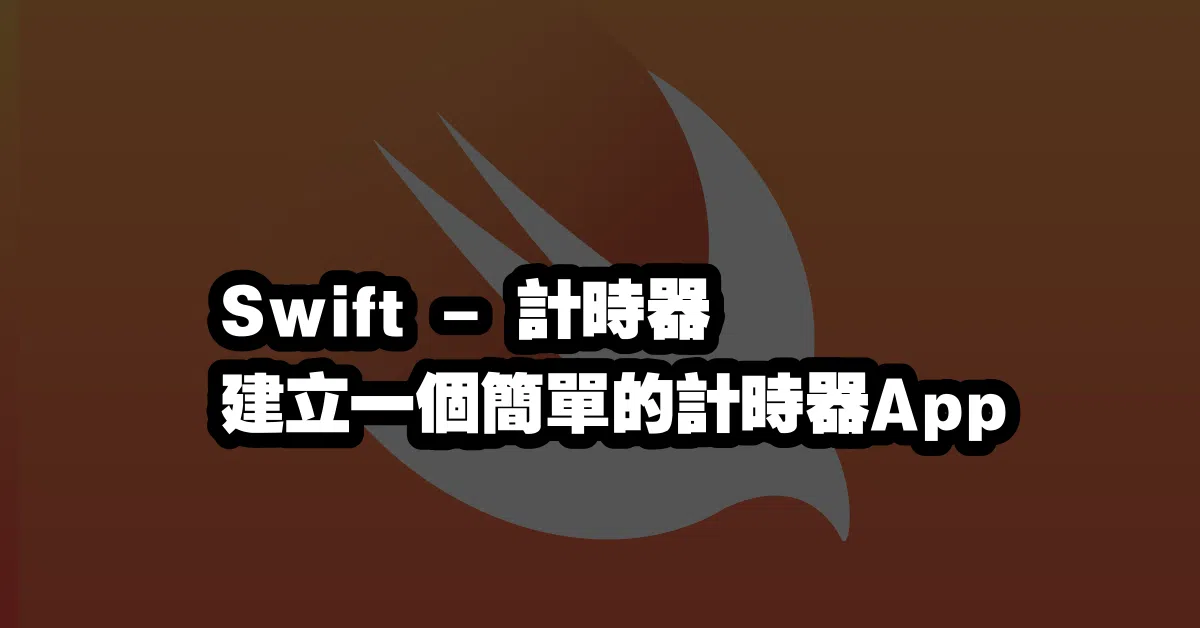# Swift – 計時器 🕰️ 建立一個簡單的計時器App

## 建立計時器

``````let timer = Timer.scheduledTimer(withTimeInterval: 1.0, repeats: true) { timer in
// 在這裡放置要執行的函式
}
``````

## 計算時間

``````let startTime = Date.timeIntervalSinceReferenceDate
``````

``````let currentTime = Date.timeIntervalSinceReferenceDate
let elapsedTime = currentTime - startTime
``````

``````let hours = Int(elapsedTime / 3600)
let minutes = Int((elapsedTime.truncatingRemainder(dividingBy: 3600)) / 60)
let seconds = Int(elapsedTime.truncatingRemainder(dividingBy: 60))
``````

## 延伸閱讀本站文章Categorized in:

Tagged in:

,